# Ellison-Glaeser Index

## Definition

The Ellison-Glaeser Index (EG) is an index developed for the assessment of industrial agglomeration

## Details

The calculation process for the EG index is detailed here (the terminology and steps are adjusted versus the original publication to typical Concentration Risk applications)

### Exposures

We have N exposures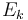$E_k$ summing up to a total exposure of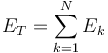$E_T = \sum^{N}_{k=1} E_{k}$

Each exposure is associated with one industry (business sector) and one geography. The total HHI index capturing single exposure concentration across the entire portfolio is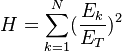$H = \sum^{N}_{k=1} (\frac{E_{k}}{E_T})^2$

We have I industries (business sectors)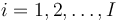$i=1,2,\ldots,I$. Each industry comprises of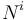$N^i$ exposures. The total exposure per industry is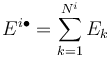$E^{i \bullet} = \sum^{N^i}_{k=1} E_{k}$

where the bullet denotes the implied summation over all areas. The fraction of each exposure within an industry is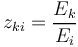$z_{ki} = \frac{E_k}{E_i}$

The HHI index within each industry is defined as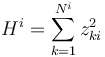$H^i = \sum^{N^i}_{k=1} z_{ki}^{2}$

The fraction of each industry as part of the total exposure is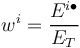$w^{i} = \frac{E^{i \bullet}}{E_T}$

The HHI index capturing concentration in the distribution of the different industry sectors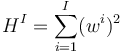$H^I = \sum^{I}_{i=1} (w^{i})^{2}$

The aggregate HHI is connected with the industry specific indexes via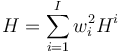$H = \sum^{I}_{i=1} w_i^2 H^{i}$

### Geographic Areas

We have M areas (countries or regions)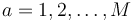$a=1,2,\ldots,M$. Each area comprises of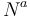$N^a$ exposures. The total exposure per area is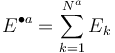$E^{\bullet a} = \sum^{N^a}_{k=1} E_{k}$

The fraction of each area as part of the total exposure is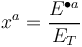$x^{a} = \frac{E^{\bullet a}}{E_T}$

The geographic concentration is captured by an HHI type metric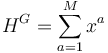$H^{G} = \sum^{M}_{a=1} x^{a}$

### Raw Concentration Metric

Within each area, an industry comprises of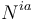$N^{ia}$ exposures, summing up to a total of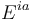$E^{ia}$ for each industry / area combination. The allocation of industry exposure to areas is given by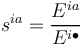$s^{ia} = \frac{E^{ia}}{E^{i \bullet}}$

An (ad-hoc) metric capturing industry exposure concentration per area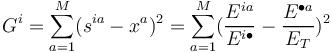$G^{i} = \sum^{M}_{a=1} (s^{ia} - x^a)^2 = \sum^{M}_{a=1} (\frac{E^{ia}}{E^{i \bullet}} - \frac{E^{\bullet a}}{E_T})^2$

This metric is zero when industrial exposure in an area as a fraction of the total in that industry is the same as the fraction of all industrial exposure in that area over all exposure.

### EG Index per industry

The EG industrial concentration index (per industry i) is given by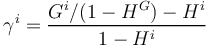$\gamma^{i} = \frac{ G^{i} / (1 - H^{G}) - H^{i}} { 1 - H^{i}}$

None

None

## Issues and Challenges

• The setup for the calculation of the EG index implies that the geographical distribution of exposures is given (i.e. it does not measure geographic distribution per se, but the relative concentration of industrial exposure given the overall geographical distribution
• The EG index is sensitive to the definition of geographical regions

## Implementations

Open Source implementations of the Ellison-Glaeser index are available in